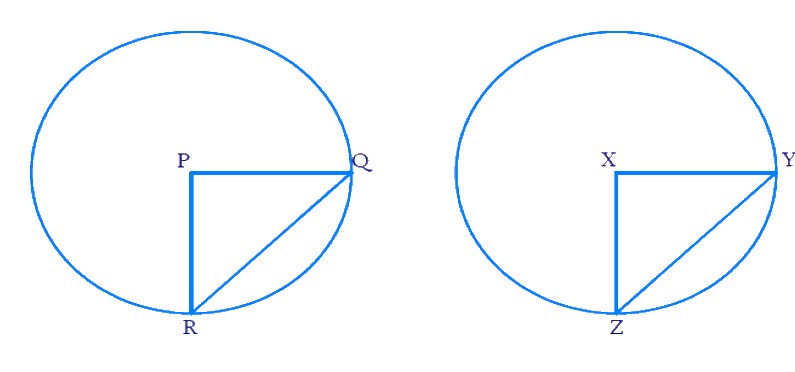# Ex.10.2 Q2 Circles Solution - NCERT Maths Class 9

## Question

Prove that if chords of congruent circles subtend equal angles at their centers, then the chords are equal.

Video Solution
Circles
Ex 10.2 | Question 2

## Text Solution

What is known?

Two circles are congruent, and their chords subtend equal angles at their centers.

What is unknown?

To find whether the chords are equal.

Reasoning:

Using equal angles at centres and the fact that circles are congruent, we prove the statement using Side-Angle-Side (SAS criteria) and Corresponding parts of congruent triangles (CPCT).

Steps:Draw chords \begin{align} {QR}\end{align} and \begin{align} {YZ}\end{align} in $$2$$ congruent circles respectively. Join the radii \begin{align} {PR}, \; {PQ} \end{align} and \begin{align} {XY, XZ }\end{align} respectively.

Given that chords subtend equal angles at centre. So\begin{align}\angle {QPR}=\angle {YXZ}\,.\end{align}

We need to prove that chords are equal. i.e \begin{align}{QR = YZ}.\end{align}

Since the circles are congruent, their radii will be equal.

\begin{align}{PR = PQ = XZ = XY}\end{align}

Consider the $$2$$ triangles \begin{align} \Delta\,{PQR}\end{align} and \begin{align} \Delta\,{XYZ}.\end{align}

\begin{align}{{PQ}} & ={{XY}} \qquad \;\; \begin{pmatrix} \text { Radii } \\ \text{are equal }\end{pmatrix} \\ {\angle {QPR}} &={\angle {YXZ}} \quad \begin{pmatrix} \! \text { Chords } \! \\ \! \text{subtend} \! \\ \! \text{equal angles} \! \\ \! \text{ at centre } \! \end{pmatrix} \\ {{PR}} &= {{XZ}} \qquad \;\; \begin{pmatrix} \text { Radii } \\ \text{are equal }\end{pmatrix} \end{align}

By SAS criteria \begin{align} \Delta { PQR}\end{align} is congruent to \begin{align} \Delta { XYZ.}\end{align}

So by CPCT (Corresponding parts of congruent triangles) \begin{align} {QR = YZ.}\end{align}

Hence proved if chords of congruent circles subtend equal angles at their centres then the chords are equal.

Video Solution
Circles
Ex 10.2 | Question 2
Learn from the best math teachers and top your exams

• Live one on one classroom and doubt clearing
• Practice worksheets in and after class for conceptual clarity
• Personalized curriculum to keep up with school